# The error term in the Prime Number Theorem

The Riemann hypothesis is equivalent to the following statement.

For every positive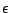, the numberof prime numbers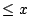is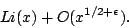Here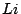is the Logarithmic integral'' function, defined by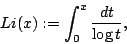the integral being evaluated in principal value in the neighbourhood of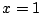.

Roughly speaking, it means that the first half of the digits of the n-th prime are those of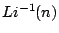.

Back to the main index for The Riemann Hypothesis.